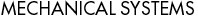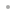MechanicalSystemsFeaturesSample ImagesContentsQ&ABuy OnlineDocumentation1. Getting Started
• Starting MechanicalSystems
• Basic 2D Model
• Basic 3D Model

2. Kinematic Modeling

• Body Objects
• Points, Vectors and Axes
• Constraint Objects
• Building the Model
• Running the Model
• Variable Management

3. Output Functions

• 2D Output Functions
• 3D Output Functions
• Plots and Graphs
• 2D Mechanism Images
• 3D Mechanism Images

4. Velocity and Acceleration

• Velocity and Acceleration Solutions
• Velocity and Acceleration Output

5. Multistage Mechanisms

• Stage Switching Constraints
• Time Switching Constraints

6. Cams and Gears

• Cam Constraints
• Gear Constraints

• Static Solutions
• Resultant Forces

8. Inverse Dynamics

• Inertia Properties
• Kinematic and Dynamic Solutions
• Friction and Damping

9. Parametric Design

• Coupled Models
• Multiple Configuration Couples
• Velocity and Acceleration Couples
• Sensitivity

10. Underconstrained Systems

• Equilibrium Configuration
• Equilibrium Velocity
• Free Acceleration
• Dynamic Motion Synthesis
• Nonholonomic Systems

11. Generalized Coordinates

• Generalized Coordinate Constraints
• Dynamic Modeling

12. Performance Improvement

• Updating Solutions
• Simplifying Expressions
• Compiling Expressions
• Coordinate Systems
• Locking Variables

13. Debugging Models

• Model Building Errors
• Failure to Converge
• Mathematical Anomalies
• Equations of Motion

Appendix: Reference Guide

• Modified Mathematica Built-in Symbols
• Listing of Mech Objects and Functions